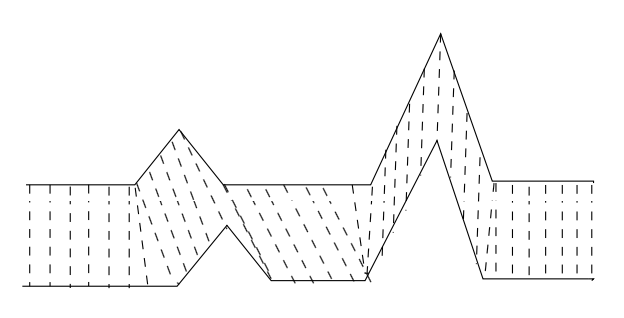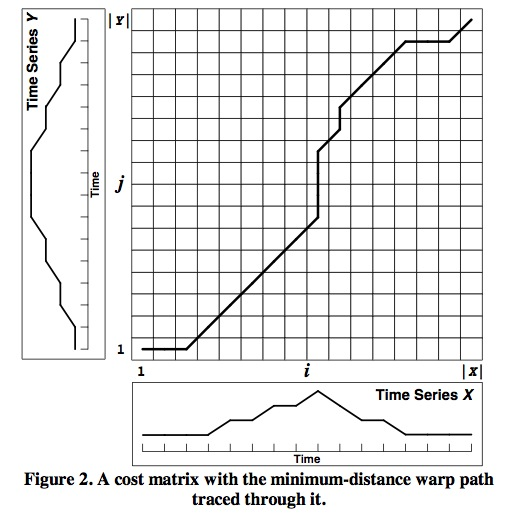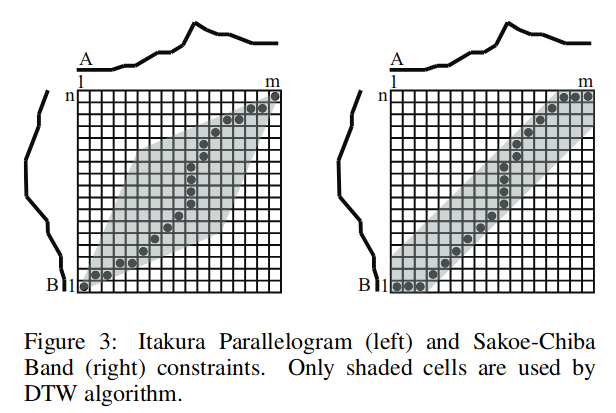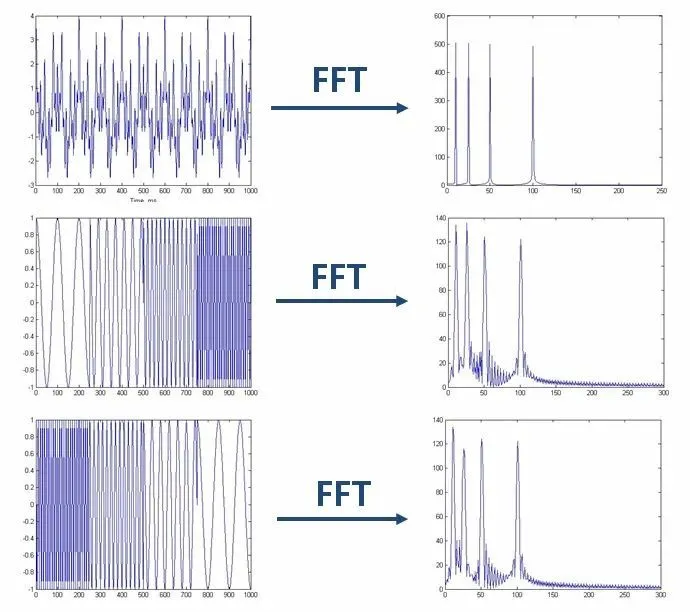2018-06-01

# 声学模型

https://zhuanlan.zhihu.com/p/23567981

# 解码器技术

$[w_1^N]^{opt}=\mathop{\arg\max}_{w_1^N,N}p(w_1^N\mid x_1^T)$

https://zhuanlan.zhihu.com/p/23648888

# 人类声音

https://wenku.baidu.com/view/6123ba2f0066f5335a8121fe.html

# 建模单元

character显然是个最粗的划分，尽管英语是表音文字，然而一个字母有多个发音，仍然是个普遍现象。

# DTW

Dynamic Time Warping是Vintsiuk于1968年提出的算法。

Taras Klymovych Vintsiuk，1939～2012，乌克兰科学家，毕业于Kyiv Polytechnic Institute。模式识别专家，语音识别领域的奠基人之一。1）边界条件：$$w_1=(1,1)$$和$$w_k=(m,n)$$。任何一种语音的发音快慢都有可能变化，但是其各部分的先后次序不可能改变，因此所选的路径必定是从左下角出发，在右上角结束。

2）连续性：如果$$w_{k-1}= (a’, b’)$$，那么对于路径的下一个点$$w_k=(a, b)$$需要满足$$(a-a’) \le 1$$和$$(b-b’) \le 1$$。也就是不可能跨过某个点去匹配，只能和自己相邻的点对齐。这样可以保证R和T中的每个坐标都在W中出现。

3）单调性：如果$$w_{k-1}= (a’, b’)$$，那么对于路径的下一个点$$w_k=(a, b)$$需要满足$$0\le (a-a’)$$和$$0\le (b-b’)$$。这限制W上面的点必须是随着时间单调进行的。以保证图1中的虚线不会相交。

$D(i,j)=Dist(i,j)+\min(D(i-1,j),D(i,j-1),D(i-1,j-1)), D(1,1) = 0$

DTW实例的具体计算过程可参见：DTW的缺点：

1.运算量大；

2.识别性能过分依赖于端点检测；

3.太依赖于说话人的原来发音；

4.不能对样本作动态训练；

5.没有充分利用语音信号的时序动态特性；

DTW适合于特定人基元较小的场合，多用于孤立词识别；

http://blog.csdn.net/zouxy09/article/details/9140207

https://blog.csdn.net/raym0ndkwan/article/details/45614813

DTW动态时间规整

http://www.cnblogs.com/luxiaoxun/archive/2013/05/09/3069036.html

Dynamic Time Warping动态时间规整算法

https://zhuanlan.zhihu.com/p/39450321

https://blog.csdn.net/songbinxu/article/details/86660136

https://zhuanlan.zhihu.com/p/72453060

DTW算法详解

https://mp.weixin.qq.com/s/pr-dUGaY-JxgUiu8HK5hqQ

# Spectrogram

## Window function

Fourier transform研究的是整个时间域和频率域的关系。因此它只能获取一段信号总体上包含哪些频率的成分，却对各成分出现的时刻并无所知。https://en.wikipedia.org/wiki/Window_function

https://mp.weixin.qq.com/s/84f-HbQSvGPr2tD9EpdbVw

https://mp.weixin.qq.com/s/6F0cFHsHRasxNVlKty8GDQ